## Tangential Angle

For a Plane Curve, the tangential angle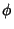is defined by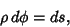(1)

where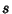is the Arc Length and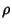is the Radius of Curvature. The tangential angle is therefore given by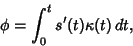(2)

where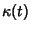is the Curvature. For a plane curve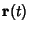, the tangential angle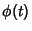can also be defined by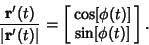(3)

Gray (1993) callsthe Turning Angle instead of the tangential angle.

Gray, A. The Turning Angle.'' §1.6 in Modern Differential Geometry of Curves and Surfaces. Boca Raton, FL: CRC Press, pp. 13-14, 1993.